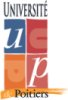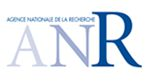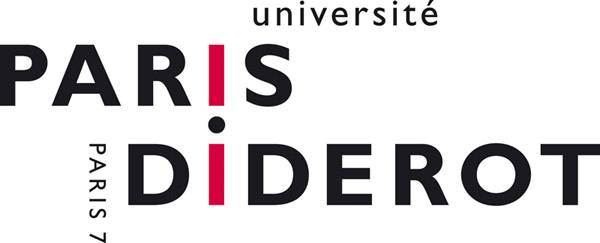## Géométrie et représentations des algèbres de Cherednik, et catégorie $$\mathcal{O}$$ « GERCHER »

Programme blanc « ANR-2010-BLAN-110-02 »# Conférence « Théorie des Représentations »

## 12-16 janvier 2015 / 12-16 January 2015 Bâtiment Sophie Germain, Université Paris Diderot, Paris, France

 Organisateurs / Organizers
 Informations Pratiques / Useful Information

 Orateurs / Speakers
 R. Bezrukavnikov P. Boalch A. Braverman A. Brochier O. Dudas P. Etingof S. Griffeth B. Ion J. Kamnitzer V. Mazorchuk I. Mirkovic A. Oblomkov G. Röhrle O. Schiffmann M. Shimozono C. Stroppel L. Topley

Programme

Les exposés auront lieu dans amphi Turing (bâtiment Sophie Germain) les 12, 13, 14 janvier
et dans amphi Gouges 2 (bâtiment Olympe-de-Gouges) les 15 et 16 janvier.

The talks will be held in amphi Turing (bâtiment Sophie Germain) on 12, 13, 14 January,
and in amphi Gouges 2 (bâtiment Olympe-de-Gouges) on 15 and 16 January.

Lundi / Monday 12Mardi / Tuesday 13Mercredi / Wednesday 14Jeudi / Thursday 15 Vendredi / Friday 16
9h15-10h30 Mazorchuk Etingof Shlmozono Bezrukavnikov Oblomkov
10h30-11h Pause café / Coffee break Pause café / Coffee break
(salle 1005, Bâtiment Sophie Germain)
11h-12h15 Schiffmann Kamnitzer Ion Brochier Griffeth
14h-15h15 Stroppel Dudas Braverman Boalch
15h15-15h45 Pause café / Coffee break Pause café / Coffee break
(salle 1005, Bâtiment Sophie Germain)
15h45-17h Röhrle Mirkovic Topley

Titres et résumés des exposés / Titles and abstracts of the talks

• Roman Bezrukavnikov
Coherent sheaves on the Hilbert scheme and perverse sheaves on spaces of stable pairs
I will report on joint works in progress with Andrei Okounkov and Galyna Dobrovolska. The former leads to t-structures on the derived category of coherent sheaves on the Hilbert scheme of points on the plane, related to modular representations of the rational Cherednik algebra, quantum cohomology etc. The latter us devoted to realizing the hearts of these t-structures as full subcategories in the category of perverse sheaves on the space of stable pairs on an elliptic curve.
• Philip Boalch
Non-perturbative symplectic geometry and non-commutative algebras
Just as a Lie group is a multiplicative version of a Lie algebra, there is a class of holomorphic symplectic varieties which are precise multiplicative versions of simpler ("additive") symplectic varieties. In this theory the role of the exponential map is played by the Riemann-Hilbert map, or its extension to the irregular case. I'll describe some examples, and show how a new theory of multiplicative quiver varieties emerges. Then I will recall how certain (multiplicative) symplectic quotients lead to noncommutative algebras and thereby construct the fission algebras (which are new multiplicative analogues of deformed preprojective algebras).
• Alexander Braverman
Symplectic duality, the Coulomb branch and the affine Grassmannian (joint work in progress with M.Finkelberg and H.Nakajima)
To a (reductive) group G and a symplectic representation M of G, physicists associate an (N=4) super-symmetric gauge theory in 3 dimensions. Any such theory is supposed to give rise to two (affine) "singular symplectic" moduli spaces - the Higgs branch and the Coulomb branch. These spaces are related by a certain mysterious "symplectic duality" (also called Gale duality) studied in mathematical literature by many authors.
While the Higgs branch is easy to define (usually, it is just the symplectic reduction of M by G), the Coulomb branch has no mathematically rigorous definition.The purpose of the talk will be to suggest such a definition in the case when M is of the form V$$\oplus$$ V* for some representation V of G, and to show that in many examples it gives the right answer. The suggestion is based on the geometry of the affine Grassmannian of G.
Topological field theories and quantum D-modules
The goal of this talk is to describe a topological construction of a certain quantum version of the category of D-modules on a reductive algebraic group G, and of its equivariant version. The latter is, at least conjecturally, closely related to the category of modules over the double affine Hecke algebra. More precisely, these categories are obtained as the value of a certain (partially defined) 4-dimensional topological field theory (TFT) on the punctured and the closed torus respectively. This TFT is constructed from the braided tensor category of modules over the quantum group of G. More generalluy, our main result is an explicit construction, in the framework of factorization homology, of the 2-dimensional part of a 4-dimensional TFT constructed from any ribbon tensor category. Time permitting I will discuss some applications of this formalism to quantization of character varieties, Witten-Reshetikhin-Turaev theory, and the so-called AJ conjecture relating the coloured Jones polynomial and the A-polynomial of a link. This is a joint work with David Ben-Zvi and David jordan.
• Olivier Dudas
Projective modules in the cohomology of Deligne-Lusztig varieties
• Pavel Etingof
Non-integral tensor powers
Let $$t$$ be a complex number, and $$V$$ a complex vector space. I will explain how to define the tensor product $$V^{\otimes t}$$. This can be done canonically if we fix a nonzero vector in $$V$$. However, the result is not a vector space but rather an (ind-)object in the tensor category $${\rm Rep}(S_t)$$, defined by P. Deligne as an interpolation of the representation category of the symmetric group $$S_n$$ to complex values of $$n$$. This category is semisimple abelian for $$t\notin\mathbf{Z}_+$$, but only Karoubian (=idempotent complete) for $$t\in\mathbf{Z}_+$$, in which case it projects onto the usual representation category of $$S_n$$. I will recall the definition of the category $${\rm Rep}(S_t)$$, and explain how Schur-Weyl duality works in this category when $$t\notin\mathbf{Z}_+$$. Then I will explain what happens at nonnegative integer $$t$$, which is more subtle and is due to Inna Entova-Aizenbud, and indicate possible applications.
• Stephen Griffeth
Eigenvalues of monodromy for parabolic restrictions of Cherednik algebra modules
This is a report on joint work with Armin Gusenbauer, Daniel Juteau, and Martina Lanini. Bezrukavnikov-Etingof discovered that modules in category O for the rational Cherednik algebra of a complex reflection group restrict to D-modules on each stratum of the reflection representation of the group. Category O is a quasi-hereditary cover of the category of representations of the finite Hecke algebra, with maps between standard modules delimited by a well-known ordering usually referred to as the "c-ordering". In certain cases, such as for the symmetric group, a coarser order combining dominance order and cores of partitions is known to wotk, giving much more detailed information about the possible maps between standard objects. We explain how the calculation of the residues of the connections studied by Bezrukavnikov-Etingof may be used to coarsen the c-ordering for arbitrary groups in such a way that one obtains versions of dominance order and cores for all reflection groups.
• Bogdan Ion
BGG reciprocity for current algebras
Current algebras are special maximal parabolic subalgebras of affine Lie algebras. In the case of untwisted affine Lie algebras they are isomorphic to the tensor product of a finite dimensional simple Lie algebra and the ring pf polynomials in one variable. The category of finite dimensional representations of current algebras is not semisimple. In 2011 Chari et al. have conjectured a version of the BGG reciprocity in this context, which connects simple finite dimensional representations, their projective covers, and standard modules. I will present a proof of this conjecture based on a connection with Macdonald theory (joint work with V. Chari).
• Joel Kamnitzer
K-theoretic quantum geometric Satake
The geometric Satake corresponds relates morphism spaces between representations to homology of Steinberg-like'' varieties constructed using the affine Grassmannian. We formulate a quantum group version of geometric Satake, where homology is replaced with equivariant K-theory. We prove this conjecture in type A.
• Volodymyr Mazorchuk
Simple fiat 2-categories and the center
The aim of this talk is to explain classification of simple fiat 2-categories and describe some parts of the 2-representation theory for such categories. In particular, we will try to explain why, from this point of view, it is difficult to derive information on surjectivity of the action of the center.
• Ivan Mirkovic
Local spaces and loop Grassmannians
The notion of a "local space" over a curve is a version of the fundamental structure of a factorization space introduced and studied by Beilinson and Drinfeld. The change of emphases leads to new constructions. The main example will be generalizations of loop Grassmannians.
• Alexei Oblomkov
Cohomology of the homogeneous Hitchin fibers and representation theory of Cherednik algebras
The talk is based on the joint work with Z. Yun.
I will explain a geometric construction of an action of the rational Cherednik algebra on the cohomology of the homogeneous Hitchin fiber for the Hitchin system on the weighted projective line. The perverse and cohomological fibrations on the cohomology have a natural representation theoretic interpretation which will be explained. In type $$A$$ case, the ring structure of the cohomology will be presented.
• Gerhard Röhrle
Equivariant K-theory of generalized Steinberg varieties
We discuss the equivariant K-groups of a family of generalized Steinberg varieties that interpolates between the Steinberg variety of a reductive, complex algebraic group and its nilpotent cone in terms of the extended affine Hecke algebra and double cosets in the extended affine Weyl group. As an application, we use this description to define Kazhdan-Lusztig "bar" involutions and Kazhdan-Lusztig bases for these equivariant K-groups.
This is a report on joint work with J.M. Douglass.
• Olivier Schiffmann
Cohomology of the moduli spaces of stable Higgs bundles
We give a closed formula for the number of absolutely indecomposable vector bundles of fixed rank and degree, over a smooth projective curve defined over a finite field. We then show that this same number computes the Poincare polynomial of the moduli space of stable Higgs bundles (of same rank and degree) over the same curve. We propose analogues, in this context, of the conjectures of Kac in the setting of quivers and Kac-Moody algebras.
• Mark Shimozono
Conjectures on the Quantum K-theory of flag varieties and K-theory of affine Grassmannians
• Catherina Stroppel
Coideal subalgebras, categorification and finite dimensional representations
Given a reductive Lie algebra and an involution I, the fixed point Lie algebra is a reductive subalgebra imbedded into the original Lie algebra. Unfortunately this does not lift to the quantum level. However, there is a coideal subalgebra which can be viewed as a quantization of the fixed point Lie algebra. In this talk we will study a particular example of such a construction, address the problem of finite dimensional representations and finally describe a categorification.
• Lewis Topley
Small modules for reduced enveloping algebras
The dimensions of modules for a reduced enveloping algebras of a Lie algebra of a reductive group over a field of positive characteristic are known to be bounded below by a number depending upon the dimension of the coadjoint orbit of the chosen p-character. In the past year this lower bound has been shown to be best possible, provided the characteristic of the underlying field is large enough. In this talk I shall describe a work in progress which attempts to construct the modules which achieve this lower bound as induced modules.